Methods and formulas for variance ratios in confidence intervals in Nested Gage R&R Study

Select the method or formula of your choice.

Confidence interval for the ratio of the repeatability variance and the total variance

There are two possible calculation methods. Minitab first calculates the bounds using the modified large-sample (MLS) method. If certain conditions are not met during the calculations, then Minitab uses the Satterthwaite approximation. To calculate the one-sided confidence bounds, replace α/2 with α in H and G.

MLS method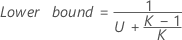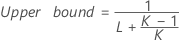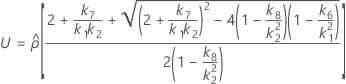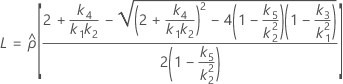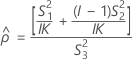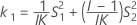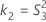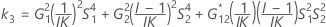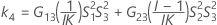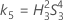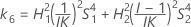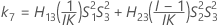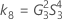The two conditions for the existence of the lower and the upper bounds using the MLS method are: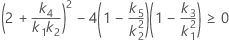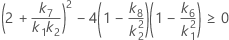If the two conditions are not satisfied, Minitab cannot use this method to construct the lower and upper bounds. Minitab will use the Satterthwaite approximation to calculate the lower and upper bounds.

Satterthwaite approximation

The formulas for the lower and upper bounds still hold except L and U are defined as follows: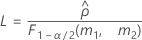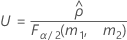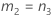Notation

TermDescription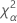the α * 100 percentile of the chi-square distribution with nq degrees of freedom
Jthe number of operators
Ithe number of parts
Kthe number of replicates

Confidence interval for the ratio of the reproducibility variance and the total variance

There are two possible calculation methods. First, Minitab calculates the bounds using the modified large-sample (MLS) method. If certain conditions are not met during the calculations, then Minitab uses an alternate approximation. To calculate the one-sided confidence bounds, replace α/2 with α in H and G.

MLS method

The lower and upper bounds for an approximate (1–α) * 100% confidence interval are calculated by solving quadratic equations.

Lower boundUpper boundSecond method

If B2– 4AC < 0, there is no solution to the quadratic equation. In this case, Minitab uses the second method to estimate the confidence intervals. The lower and upper bounds for an approximate (1–α) * 100% confidence interval are calculated as follows:Notation

TermDescriptionthe α * 100 percentile of the chi-square distribution with nq degrees of freedom
Jthe number of operators
Ithe number of parts
Kthe number of replicates

Confidence interval for the ratio of the part variance and the total variance

There are two possible calculation methods. First, Minitab calculates the bounds using the modified large-sample (MLS) method. If certain conditions are not met during the calculations, then Minitab uses an alternate approximation. Replace α/2 with α in H and G to calculate the one-sided confidence bounds.

MLS method

The lower and upper bounds for an approximate (1–α) * 100% confidence interval are calculated by solving quadratic equations.
Lower boundUpper boundSecond method

If B2– 4AC < 0, there is no solution to the quadratic equation. In this case, Minitab uses the second method to estimate the confidence intervals. The lower and upper bounds for an approximate (1–α) * 100% confidence interval are calculated as follows:Notation

TermDescriptionthe α * 100 percentile of the chi-square distribution with nq degrees of freedom
Jthe number of operators
Ithe number of parts
Kthe number of replicates

Confidence interval for the ratio of the gage variance and the total variance

Lower bound = 1 – (lower bound of the CI for the ratio of the part variance and the total variance)

Upper bound = 1 – (upper bound of the CI for the ratio of the part variance and the total variance)

By using this site you agree to the use of cookies for analytics and personalized content.  Read our policy